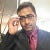# Rank of Matrix

The rank of the matrix is defined as “Largest order of any non-vanishing minor of the matrix”. It is denoted by R(A).

Example:

Question: Find the Rank of the matrix

Define some alias to write mathematic expressions easily and it’s also easy to understand.

--

--

# Python reduce() Function

The function reduce(function_name, seq) continually applies the function function_name() to the sequence seq. It returns a single value.

If seq = [ a1, a2, a3, … , an ], calling reduce(func, seq) works like this: At first the first two elements of seq will be applied to func, i.e. function_name(a1,a2) The list on which reduce() works looks now like this: [ function_name(a1, a2), a3, … , an ] In the next step func will be applied on the previous result and the third element of the list, i.e. func(func(a1, a2),a3) The list looks like this now: [ function_name(function_name(a1, a2),a3), … , an ] Continue like this until just one element is left and return this element as the result of reduce() We illustrate this process in the following example:

--

--

# Python filter() Function

The function filter(function_name, list) offers an elegant way to filter out all the elements of a list, for which the function function_name returns True. The function filter(f,l) needs a function f as its first argument. f returns a Boolean value, i.e. either True or False. This function will be applied to every element of the list l. Only if f returns True will the element of the list be included in the result list.

--

--

# Python map() Function

The advantage of the lambda operator can be seen when it is used in combination with the map() function. map() is a function with two arguments:

r = map(function_name , seq)

The first argument function_nameis the name of a function and the second a sequence (e.g. a list) seq. map() applies the function function_name to all the elements of the sequence seq. It returns a new list with the elements changed by funcfunction_name.